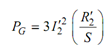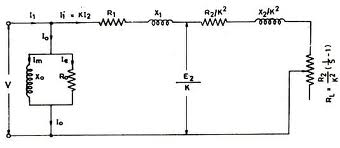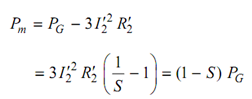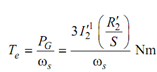## Air Gap Power - Torque and Output Power Assignment Help

Assignment Help: >> Induction Motors - Air Gap Power - Torque and Output Power

Air Gap Power, Torque and Output Power:

As illustrated in Figure, the power crossing the terminals 1-2 is the electrical power input per phase minus the stator copper loss and iron loss. It is the power transferred from the stator to the rotor via the air gap magnetic field. The PG is the three-phase value and known as air gap power.

It is apparent from the equivalent circuit thatPower across air gap = Rotor copper loss /SlipFigure: Equivalent Circuit of Induction Motor

Therefore mechanical power output (Gross)Rotor speed is

ωr  = (1 - S ) ωs  rad (mech)/sec

Then the electromagnetic torque developed is given by

(1 - S ) ωs Te  = Pm  = (1 - S ) PG

orThe net mechanical power output and torque are attain by subtracting losses-windage, friction and stray load loss.